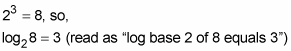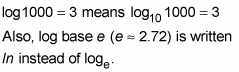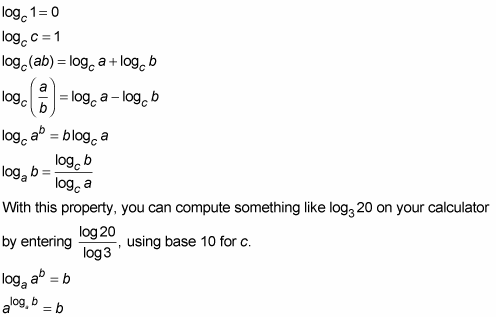##### Differential Equations For DummiesYou may come across logarithms in your calculus work. A logarithm is just a different way of expressing an exponential relationship between numbers. For instance,These two equations say precisely the same thing. You could think of the exponential equation as the normal, English way of expressing the mathematical connection between 2, 3, and 8 and the logarithmic equation as the Greek or Latin way of expressing the very same connection.

The base of a logarithm can be any number greater than zero, other than 1, and by convention, if the base is 10, you don’t write it. For example,You should know the following logarithm properties: